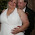Bond with James: Three-Variable Formula Triangle

## Saturday, February 16, 2013

### Three-Variable Formula Triangle

Below is a picture of a formula triangle that works for three-variable equations, such as: speed, density, or acceleration. My first year I taught IPC (Integrated Physics and Chemistry). As a biology major, I was unprepared  to teach math to students in my class and struggled to assist students who did not understand how to manipulate mathematical equations.

Apparently in Texas, the three-variable equation triangle is, or at least was, a major tool in helping students solve problems. I taught it in addition to teaching how to manipulate equations. Of course, with the new state exam in Texas, the STAAR EOC, only a few of the equations are able to use this tool.

Okay...let me stop rambling and get on with the explanation. First, you need a three-variable equation as previously mentioned. The picture below illustrates the speed equation

speed = distance
time

The variable on the left of the equal sign goes in the bottom left quadrant of the triangle. The variable in the numerator goes in the top quadrant, and the variable in the denominator goes in the bottom right quadrant.

Horizontal line = division  ; vertical line = multiplication

The flaps on this particular foldable are not really needed if students know how to work the triangle. However, you could have students add the units and/or definition of each variable to the outside of each flap.

How it works...
....cover up the variable you are looking for. Let's say you wanted to find distance. You would use your finger (or whatever) to cover up the D. That leaves S (speed) and T (time). In this case, you would multiple the two variables (since the vertical line means multiplication).

If you were looking for Time (T), you would cover up the T leaving D (distance) and S (speed). In this case, you would divide distance by speed to solve for time.

Again, this will work for any three-variable equation similar to the speed equation shown above. Most of the equations required for the Chemistry and Physics STAAR EOCs cannot utilize this particular tool, however.

1.Thanks for the update. I plan to use this with my kiddos next week.

2.Rae
Mindful Rambles

3.WOW!! I will have to try that with my son. They are working on it right now in math!

Thank you for donating to my 200 follower giveaway!

Cindy (All Things Teachery)
http://cynthialusk.blogapot.com

4.This is such a great manipulative - and just in time for me to teach the Speed Equation to my 8th graders tomorrow! Thanks so much!

5.